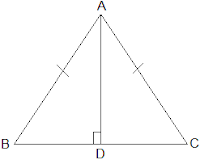Newbie

# AD is an altitude of an isosceles triangle ABC in which AB = AC. Show that (i) AD bisects BC (ii) AD bisects A. Q.2

• 0

Find the simplest solution of ncert class 9th of chapter triangles . Please help me to solve the best solution of exercise 7.3 question number 2 . How i solve this question in a simplest and easiest way please help me to find out this question. AD is an altitude of an isosceles triangle ABC in which AB = AC. Show that (i) AD bisects BC (ii) AD bisects A.

Share

1. It is given that AD is an altitude and AB = AC. The diagram is as follows:(i) In ΔABD and ΔACD,

AB = AC (It is given in the question)

∴ ΔABD ΔACD by RHS congruence condition.

Now, by the rule of CPCT,

BD = CD.